# S5E2 - Boyle's Law, Charles' Law, and Avogadro's Law

## The Gas Laws

Today we're going to talk about the three gas laws (in order): Boyle's Law, Charles Law, and Avogadro's Law.

Then, we'll move on to the Combined Gas Law and the Ideal Gas Law.

First, the three gas laws...

=====

## Boyle's Law

Boyle's Law PV = k  ,  at constant temperature (T)
P  =  pressure of the gas
V  =  volume of the gas
k  =  "some constant" (value unimportant)

balloon scenario = squeezing a balloon decreases its volume...

➞ so PV = k  (as P, V )  -- they are inversely proportional.

Boyle's Law Calculations will always involve a "situation 1" and a "situation 2." So this is the formula to remember: P1V1 = P2V2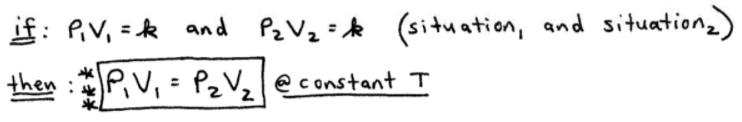ex:  Consider a 1.53L sample of SO2(g) at a pressure of 5.6 x 103 Pa.  If the pressure is increased to 1.5 x 104 Pa at a constant temperature, what's the new volume of the gas?

➞ start with Boyle's Law, then "plug and chug" so to speak...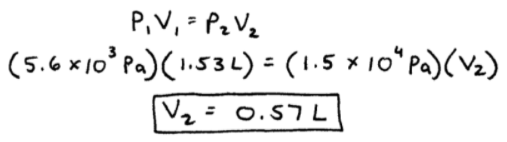The above image nicely shows the flow of how to do the problem:

P1V1 = P2V2

( 5.6 x 103 Pa ) (1.53 L )  =  ( 5.6 x 104 Pa ) ( V2 )

V2 = 0.57 L

=====

## Charles' Law

Charles' Law ➞ V / T = k  ,  at constant pressure (P)
V  =  volume of the gas
T  =  temperature of the gas (in Kelvin!)
k  =  "some constant" (value unimportant)

➞ balloon scenario = as you increase the temperature of the gas in a balloon, you also increase the gas' volume.

➞ think of a limp hot air balloon -- what happens when heat (fire torch) is turned on? Yes, the balloon expands.

➞ so V / T = k  (as T, V )  -- they are directly proportional.

Charles' Law Calculations will always involve a "situation 1" and a "situation 2." So this is the formula to remember: V1 / T1  =  V2 / T2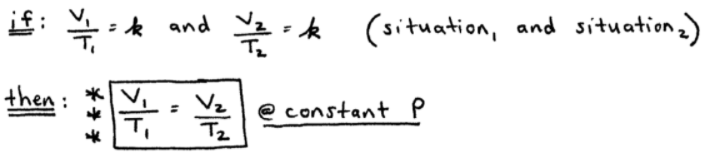ex:  A sample of gas at 15°C and 1atm has a volume of 2.58L.  What volume will this gas occupy at 38°C and 1atm?

➞ convert Celsius to Kelvin temperature, then plug in values...

15 + 273 = 288K
38 + 273 = 311KHere it is again:

V1 / T1 = V2 / T2

( 2.58 L ) / (288 K )  =  ( V2 ) / ( 311 K )

V2 = 2.79 L

=====

Avogadro's Law ➞ V / n = k  ,  at constant pressure (P) and temperature (T)
V  =  volume of the gas
n  =  number of moles of the gas
k  =  "some constant" (value unimportant)

➞ for a gas at constant temperature and pressure, the volume is directly proportional to the number of moles of the gas.

➞ so V / n = k  (as n, V )  -- they are directly proportional.

Avogadro's Law Calculations will always involve a "situation 1" and a "situation 2." So this is the formula to remember: V1 / n1  =  V2 / n2ex:  Suppose you have 12.2 L of O2 gas, containing 0.50 mol at a pressure of 1 atm and temperature of 25°C.

If all of this O2 were converted to ozone, O3, at the same temperature and pressure, what would be the volume of the ozone?

-- okay, the question is asking for the volume of O3.

-- assuming this is V2, we need to calculate n2 for O3 (not O2) before applying Avogadro's Law. So,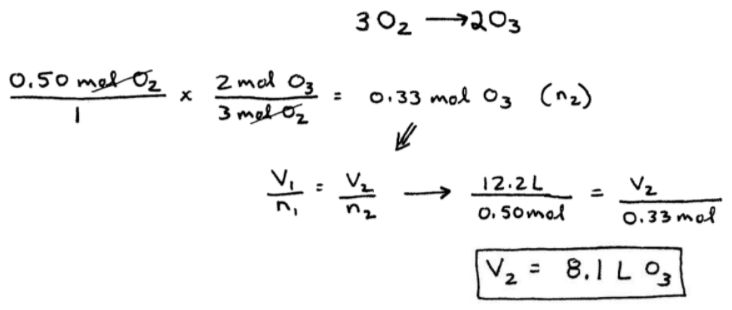Now, putting all 3 laws together to get the combined gas law and the ideal gas law.

=====

## The Combined Gas Law

Combined Gas Law = take a look at the image below. In each of the 3 gas laws, the P's and V's are in the numerator, while the n's and T's are in the denominator.

Putting them all together, we get:   P1V1 / n1T1  =  P2V2 / n2T2

Or, PV / nT  =  k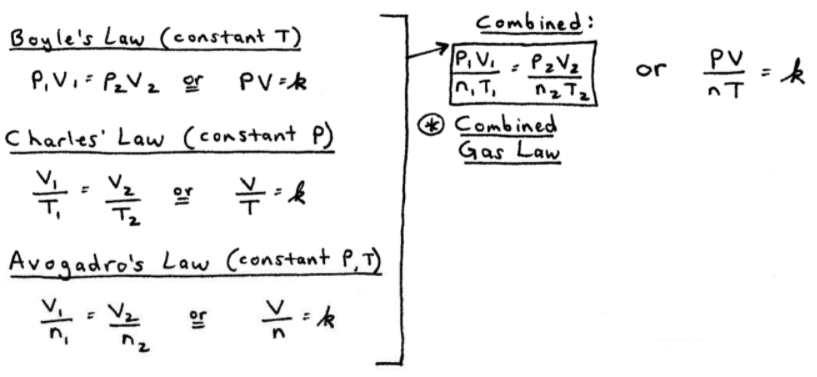-----

Take a look at the "far right" equation in the image above:  PV / nT  = k

If we define "k" as the universal gas constant (R), we get: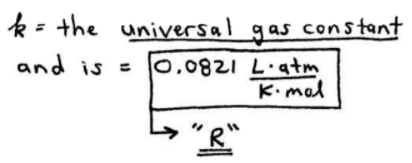Alright, now we just need to substitute R in for k to get the Ideal Gas Law.

=====

## The Ideal Gas Law

The Ideal Gas Law does NOT have a "situation 1" and "situation 2," so there's no subscripts 1 and 2.

### PV = nRTUnlike Boyle's Law, Charles' Law, Avogadro's Law, and the Combined Gas Law, the Ideal Gas Law contains the universal gas constant, "R".

And "R" has specific units!

The units of "R" force us to have:

-  Pressure in atm
-  Volume in liters
-  Moles in moles (duh)
-  Temperature in Kelvin

=====

In my next post on this topic, we'll do a TON of gas law and gas stoichiometry practice problems together.

So stick around and check out my next video post from SECTION 5 - Gases.

We'll go over a bunch of Ideal Gas Law Examples and Gas Law Practice Problems...